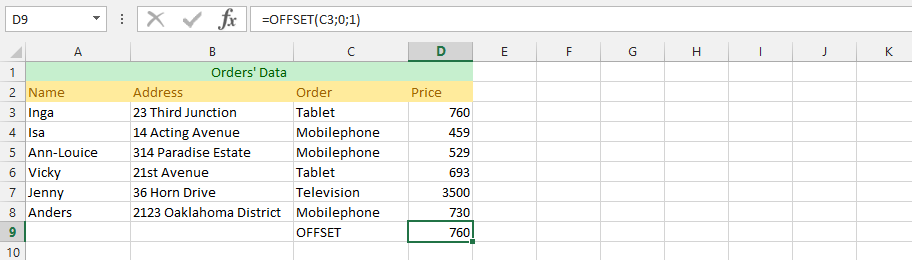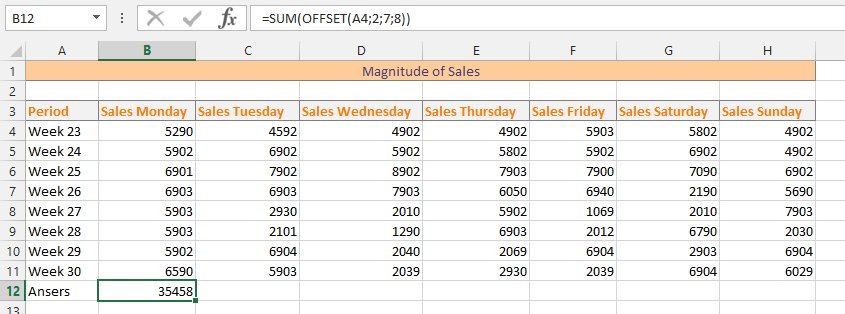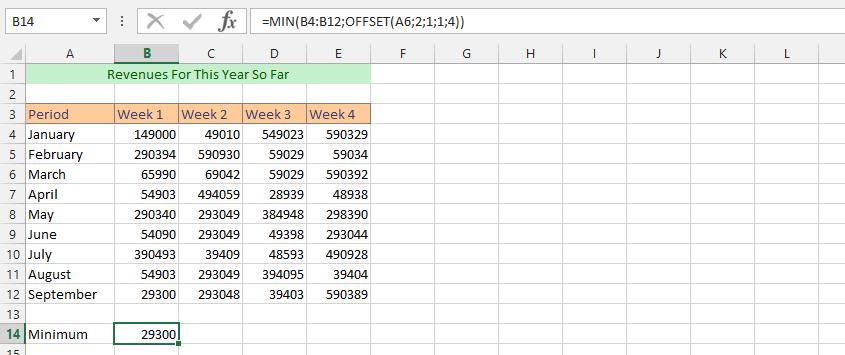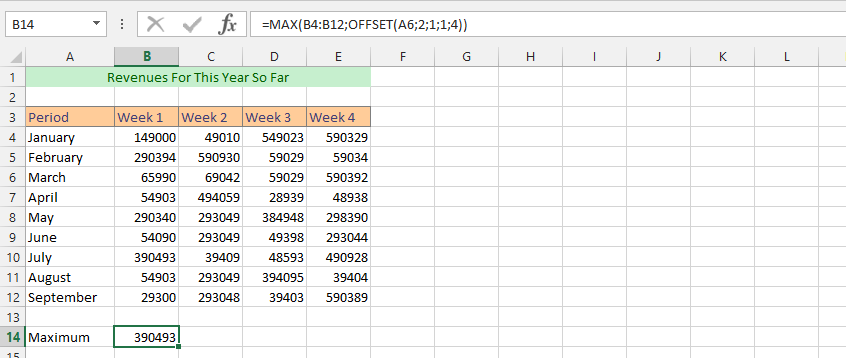### Ways that OFFSET Function Is Absolutely Useful in Business

In this tutorial you will learn how to use the offset function. The OFFSET function is a very useful benefit that comes with the Microsoft Excel, and there are more than 10 different circumstances, where it might be needed. However, we provide you with 10 different examples and formulas where the function is extremely useful in business. This is a function that would return the range of cells, using the specified numbers of both columns and rows along with the beginning of the range, as it has been specified by the users. This is because the users have the ability to specify a size of range that would be returned, which gives the user a strong opportunity to decide the range of his or her desire.

OFFSET is a function of Excel which allows you to select an area on the basis of selected parameters. OFFSET returns the field – a field of a sheet, or if you just pointed it yourself by mouse.

These parameters are as specified by Microsoft Excel, and are reference, followed by rows, column, and the height and finally the width. Syntax of the offset function is:

### OFFSET (reference, rows, cols, height, width)

• Reference: This is essential and required by the Microsoft Excel, as it is the initial range that needs to be offset. It is possible for it to be either one cells or more cells, depending on the desire of its users
• Rows: This is critical to the function and is the number of rows right from the beginning of that range that has been initially supplied to ace as beginning of range that would be returned.
• Cols (Columns): This is required by the function and act as the quantity of columns from the beginning, usually upper left of a range that has initially be supplied to a beginning of range that has returned.
• Height [height]: It is optional for the user, and would specify height of range that has returned. If this has been omitted, then it would automatically be returning a range at exactly the same range it has been supplied.
• Width [Width]: This is the last and optional argument that the offset function contains, and it solely specify width of a range that has returned. If this is omitted, then a range of returned value would automatically be the one the user supplied.

### Examples of OFFSET function’s formulas:

Example 1: A Simple OFFSET Usage

The order list has been established, and we’d know whom our customers are, and their orders as well as prices. However, there is a key question that we are currently asking, which is how much would a specific customer pays for the product that has been ordered. This is where OFFSET functions (and other functions of Excel) would be extremely useful. We are going to use it to find the price that would be paid for a specific product.

=OFFSET(C3,0,1)Example 2: IF and OFFSET Functions for Product List

The situation in this example is that we have a product lists, and we do not know which one would be for which, which is why we have to look for the right answer. The price has been set, but we want to know where the right value should be placed, and one of the best ways that we’d know is using both the IF and OFFSET functions simultaneously.

=OFFSET(B4<650,OFFSET(B4,0,0))Example 3: Magnitude of Sales

The business is going good, and we have different considerations about how the business has been performing on the market, but we want to know something about the business, and we believe that the OFFSET and SUM would be the perfect answer to our problem, so we decided to find the answer that seems to have deluded us for a short period of time, which is why we are looking for the necessary answers with Microsoft Excel.

=SUM(OFFSET(A4,2,7,8,))Example 4: Extracting Sales for a Specific Period with OFFSET

This is the follow-up to the previous example, and this time we have a different problem. We’d have so many sales periods, and we are looking for the sales for a period. There has been different methods that are useful for finding the same thing, but this time we believe that the OFFSET function is the perfect tool. In this formula, after we have marked all the cells that we would like to cover, we’d write the formula, and then type F2, followed by CTRL, SHIFT and close it with pressing enter on the keyboard.

(=OFFSET(A5,2,1,1,7)}Example 5: Advanced Usage of OFFSET & Match Function in Excel

In this example, we do have a business ready, and we are looking for a new way to get the business going, which is why we have decided to use the OFFSET and MATCH function to find the highly suitable and beneficial way of doing it. As the case has been for quite some time, we want to find a suitable way to find the sales for a specific period. There are other functions that could do the job, but we would prefer using the Match and OFFSET. We are using the same file as the two previous examples, and we think that the best way to do so is to clarify that it is very possible to perform these acts without compromising the information of the sales’ quotes on the weeks.

(=OFFSET(A5,MATCH(A14,A4,A11,0),1,3,8,)}Example 6: Compromises with OFFSET Formula with SUM and MATCH

This example is using the same data as previous examples, but we are going to use a different formula combining the OFFSET with SUM and MATCH in the same formula. This is to provide the opportunities to find a very supportive formula that allows us to get the right information. This allows the opportunity to sum the data for both week 28 and 29.

=SUM(OFFSET(A4,MATCH(A14,A4,A11,0),1,-2,7))Example 7: MIN and OFFSET to Get a Value

The business has been going good, and we want to know how we have performed within a period of time. For that reason, we want to know how much minimum we made within that period of time. This is because we have set a goal for ourselves that we want to perform in the first week of every month, and due to the circumstances we want to know if we made that minimum for that month. The minimum we have set is 40000 for the first week. Min and Offset functions will assess if that goal has been accomplished or not.

=MIN(B4:B12,OFFSET(A6,2,1,1,4))Example 8: Max and Offset Functions in Business Performance

The business has been going great for the last months, and we want to assess the business performance within this period of time to understand how the business has greatly been performing. This is something that would help us know if we could compensate for the time when we performed less. For this reason, we are going to use the same data as the previous one, and want to comprehend how our highest performance, and precisely how much it is.

=MAX(B4:B12,OFFSET(A6,2,1,1,4))Example 9: Last Year and This Year OFFSET and Min Function

This is a complicated example, and we are going to use this example because it might be useful for you in the long run. This example is solely based on having the last year saved in one document, and this year is current, and you would like to know the minimum performance for the previous year to compare with this year. We have decided that the business has to achieve a certain goal within a period of time. The assessments have great impact on acknowledging our performances.

=MIN(‘[Min and OFFSET Extra.xlsx]Sheet1’!\$B\$4:\$B\$15,OFFSET(‘[Min and OFFSET Extra.xlsx]Sheet1’!\$B\$6,2,1))Example 10: Follow-up on 9; OFFSET Different Spreadsheet

This example is like a follow-up on the example 9. The reason is that some people would probably ask a question, what if I rather use a different sheet than another document, which is the reason that this example would be useful for it to be one successful. The problem here is that we have our performance for 2014 ready, and we would like to use OFFSET to find a specific answer, and useful data that would clarifies the thing we are looking for, which is why we have decided to use it. We are looking for our performance for week one of June 2014, which is in another spreadsheet.

=OFFSET(Sheet1!A8,1,1,1,4)These are most commonly used Excel OFFSET formulas for business.

### More OFFSET examples:

=OFFSET(A1:B2,1,1,1,1) – the formula returns the value of cell B2

=OFFSET(A1,2,2) – returns the value in cell C3 (A1 moved by two rows and two columns down). If the cell C3 contains the value 100, the function returns a value of 100

=SUM(OFFSET(A1,2,2,5,6)) – is the total size of the area beginning in cell C3, which has a height of 5 rows and 6 columns (area =C3:H7)

This extract every fourth value

=OFFSET(\$B\$2,(ROW()-2)*4.0)

This sum sales for a specified period:

=SUM(OFFSET(\$B\$2,0,0,\$D\$2,1))

This extract every other column:

=OFFSET(\$A2,0,(COLUMN()-1)*2)

=COUNT(OFFSET(A1,2,2,5,6)) – counts cells in C3:H7 area

=AVERAGE(OFFSET(A1,2,2,5,6)) – calculates average in C3:H7 area

You will also need OFFSET function to create Dynamic Charts.

Previous articleHow To Link Objects In Excel